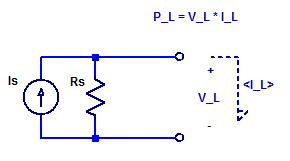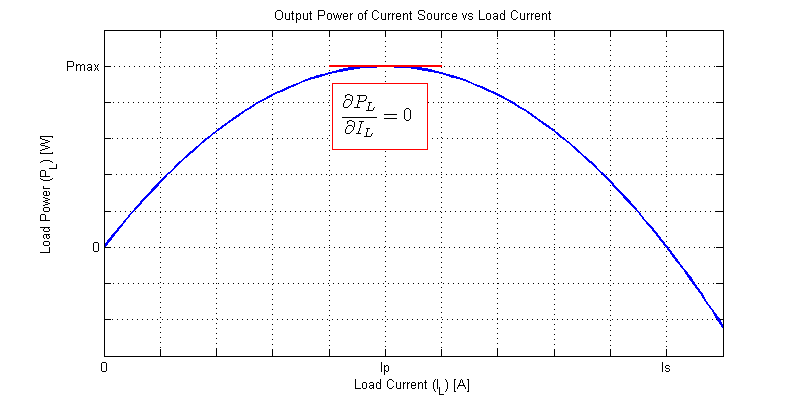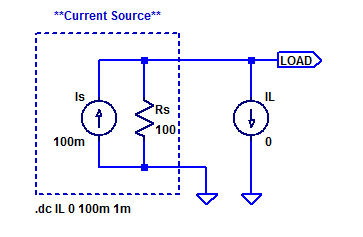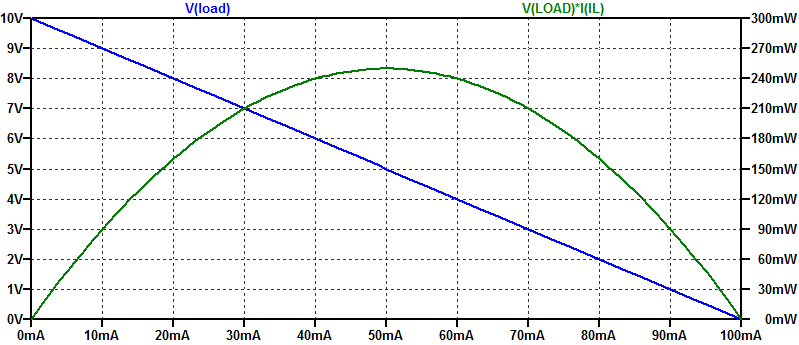# Maximum Power Point of a Current Source

While it is true an ideal current source can deliver an infinite amount of output power (at a constant current), as the output compliance voltage is infinite. A real current source has a finite output resistance which limits the maximum output voltage that can be developed across the current source. To asses the peak output power of a current source, we will develop a relation for the output voltage as a function of load current. Consider the schematic of a current source connected to some arbitrary load $$V_L$$ & $$I_L$$.KCL across the output nodes of the current source yields,

$$\dfrac{V_L}{R_s} + I_o = I_s$$

$$V_L = \left( I_s – I_o \right) R_s$$

The power consumed by the load is then simply the current-voltage product as,

\begin{align*}
P_L &= V_L I_L \\
&= \left( I_s – I_L \right) R_s I_L \\
&= R_s \left( I_sI_L – I_L^2 \right) \\
\end{align*}

The output power is a 2nd order function of load current. A normalized graph of load power versus load current when powered by a current source is shown in the figure below.At some load current $$I_P$$ the output power reaches its maximal value and then begins to decline. We can solve for the maximum power point $$P_{max}$$ as the point where the first derivative of $$P_L(I_L)$$ equals 0.

$$\dfrac{\partial P_L}{\partial I_L} = I_sR_s -2R_sI_L$$

\begin{align*}
\left| \dfrac{\partial P_L}{\partial I_L} \right|_{I_L=I_P} &= 0\\\\
0 &= I_sRs – 2R_sI_P \\\\
I_P &= \dfrac{I_s}{2} \\\\
\end{align*}

Hence, the maximum load power occurs when the load current equals $$I_s/2$$.

It should come as no surprise that a resistive load which consumes the maximum available power is a resistor of $$R_L = R_S$$.

The maximum available power is then,

\begin{align*}
P_{max} &= P_L( I_P )\\
&= R_s( I_sI_P – I_P^2 )\\
&= R_s( \dfrac{I_s^2}{2} – \dfrac{I_s^2}{4} )\\
P_{max} &= \dfrac{I_s^2R_s}{4} \\
\end{align*}

## Spice Example

Consider a current source with  the following parameters,

$$I_s = 100 \text{ [mA]}\;,\;\;\; R_s = 100 \; [\Omega]$$

A sample spice test jig is shown in the figure below.Ideal current source $$I_s$$ and shunt resistance $$R_s$$ form a  current source with output resistance $$R_S$$. An ideal current source is parametricly swept from $$0$$ mA to $$100$$ mA as a synthetic load. The results of the simulation are shown in the figure below.The open-circuit voltage of the current source is $$V_{oc} = I_s R_S = 10$$ VDC. the short-circuit current is $$I_{sc} = I_s = 100$$ mA. The maximum power point occurs at a load current of $$50$$ mA, with a corresponding peak load power of $$250$$ mW.

The maximum power point as estimated from the analysis above occurs at a load current of,

$$I_P = \dfrac{I_s}{2} = 50 \text{ [mA]}$$

With a maximum power point of,

$$P_{max} = \dfrac{I_s^2R_s}{4} = 250 \text{ [mW]}$$

## Solar Perspective

If a solar cell were to appear as a current source with shunt resistance $$R_S$$ then its short-circuit current is simply,

$$I_{SC} = I_S$$

The cells open circuit voltage is,

$$V_{OC} = I_S R_S$$

The maximum power available from the cell would be,

$$P_{max} = \dfrac{I_s^2R_s}{4} = \dfrac{V_{OC}I_{SC}}{4}$$

Hence a current source with shunt resistance has a fill factor of 0.25.

This site uses Akismet to reduce spam. Learn how your comment data is processed.